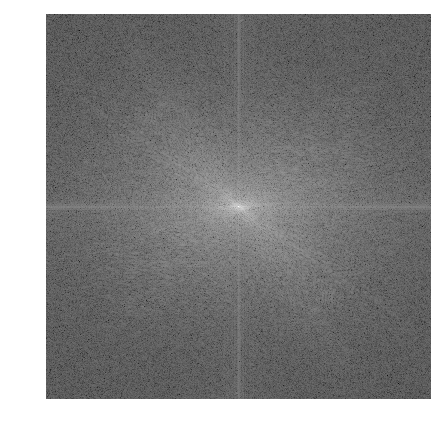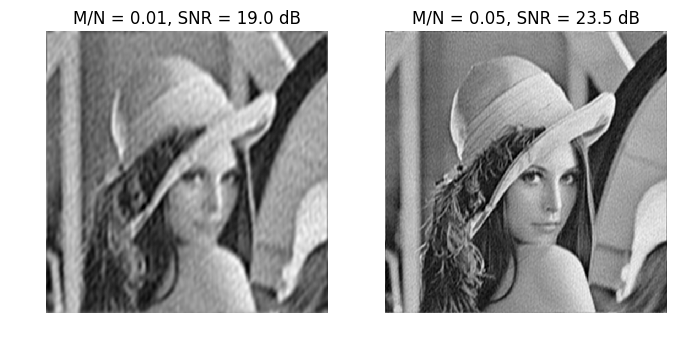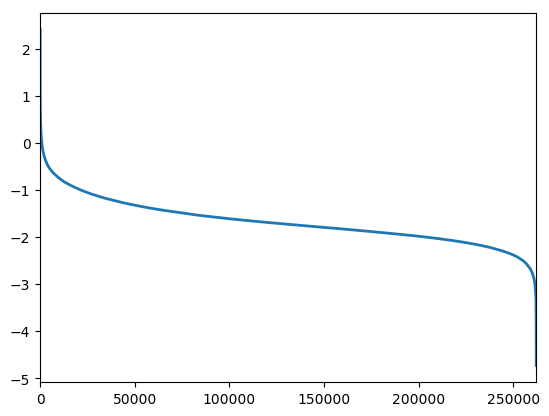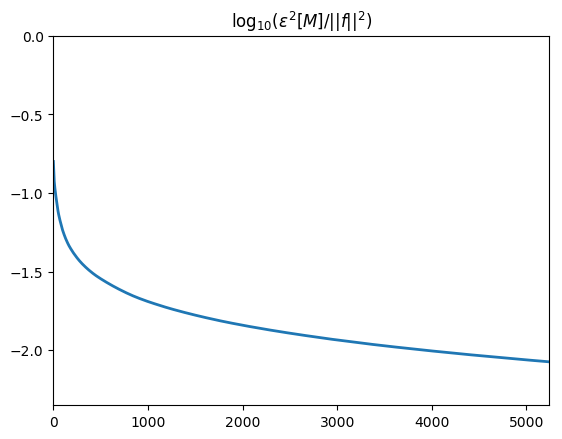# Image Approximation with Orthogonal Bases¶

Important: Please read the installation page for details about how to install the toolboxes. $\newcommand{\dotp}{\langle #1, #2 \rangle}$ $\newcommand{\enscond}{\lbrace #1, #2 \rbrace}$ $\newcommand{\pd}{ \frac{ \partial #1}{\partial #2} }$ $\newcommand{\umin}{\underset{#1}{\min}\;}$ $\newcommand{\umax}{\underset{#1}{\max}\;}$ $\newcommand{\umin}{\underset{#1}{\min}\;}$ $\newcommand{\uargmin}{\underset{#1}{argmin}\;}$ $\newcommand{\norm}{\|#1\|}$ $\newcommand{\abs}{\left|#1\right|}$ $\newcommand{\choice}{ \left\{ \begin{array}{l} #1 \end{array} \right. }$ $\newcommand{\pa}{\left(#1\right)}$ $\newcommand{\diag}{{diag}\left( #1 \right)}$ $\newcommand{\qandq}{\quad\text{and}\quad}$ $\newcommand{\qwhereq}{\quad\text{where}\quad}$ $\newcommand{\qifq}{ \quad \text{if} \quad }$ $\newcommand{\qarrq}{ \quad \Longrightarrow \quad }$ $\newcommand{\ZZ}{\mathbb{Z}}$ $\newcommand{\CC}{\mathbb{C}}$ $\newcommand{\RR}{\mathbb{R}}$ $\newcommand{\EE}{\mathbb{E}}$ $\newcommand{\Zz}{\mathcal{Z}}$ $\newcommand{\Ww}{\mathcal{W}}$ $\newcommand{\Vv}{\mathcal{V}}$ $\newcommand{\Nn}{\mathcal{N}}$ $\newcommand{\NN}{\mathcal{N}}$ $\newcommand{\Hh}{\mathcal{H}}$ $\newcommand{\Bb}{\mathcal{B}}$ $\newcommand{\Ee}{\mathcal{E}}$ $\newcommand{\Cc}{\mathcal{C}}$ $\newcommand{\Gg}{\mathcal{G}}$ $\newcommand{\Ss}{\mathcal{S}}$ $\newcommand{\Pp}{\mathcal{P}}$ $\newcommand{\Ff}{\mathcal{F}}$ $\newcommand{\Xx}{\mathcal{X}}$ $\newcommand{\Mm}{\mathcal{M}}$ $\newcommand{\Ii}{\mathcal{I}}$ $\newcommand{\Dd}{\mathcal{D}}$ $\newcommand{\Ll}{\mathcal{L}}$ $\newcommand{\Tt}{\mathcal{T}}$ $\newcommand{\si}{\sigma}$ $\newcommand{\al}{\alpha}$ $\newcommand{\la}{\lambda}$ $\newcommand{\ga}{\gamma}$ $\newcommand{\Ga}{\Gamma}$ $\newcommand{\La}{\Lambda}$ $\newcommand{\si}{\sigma}$ $\newcommand{\Si}{\Sigma}$ $\newcommand{\be}{\beta}$ $\newcommand{\de}{\delta}$ $\newcommand{\De}{\Delta}$ $\newcommand{\phi}{\varphi}$ $\newcommand{\th}{\theta}$ $\newcommand{\om}{\omega}$ $\newcommand{\Om}{\Omega}$

This numerical tour uses several orthogonal bases to perform non-linear image approximation.

In :
using PyPlot
using NtToolBox
# arequire("NtToolBox")


## Best $M$-terms Non-linear Approximation¶

This tours makes use of an orthogonal base $\Bb = \{ \psi_m \}_{m=0}^{N-1}$ of the space $\RR^N$ of the images with $N$ pixels.

The best $M$-term approximation of $f$ is obtained by a non-linear thresholding

$$f_M = \sum_{ \abs{\dotp{f}{\psi_m}}>T } \dotp{f}{\psi_m} \psi_m,$$

where the value of $T>0$ should be carefully selected so that only $M$ coefficients are not thresholded, i.e.

$$\abs{ \enscond{m}{ \abs{\dotp{f}{\psi_m}}>T } } = M.$$

The goal is to use an ortho-basis $\Bb$ so that the error $\norm{f-f_M}$ decays as fast as possible when $M$ increases, for a large class of images.

This tour studies several different orthogonal bases: Fourier, wavelets (which is at the heart of JPEG-2000), cosine, local cosine (which is at the heart of JPEG).

First we load an image of $N = n \times n$ pixels.

In :
n = 512


Display it.

In :
figure(figsize = (5,5))
imageplot(f)## Fourier Approximation¶

The discrete 2-D Fourier atoms are defined as: $$\psi_m(x) = \frac{1}{\sqrt{N}} e^{ \frac{2i\pi}{n} ( x_1 m_1 + x_2 m_2 ) },$$ where $0 \leq m_1,m_2 < n$ indexes the frequency.

The set of inner products $\{ \dotp{f}{\psi_m} \}_m$ is computed in $O(N \log(N))$ operations with the 2-D Fast Fourier Transform (FFT) algorithm (the pylab function is fft2).

Compute the Fourier transform using the FFT algorithm. Note the normalization by $1/\sqrt{N}$ to ensure orthogonality (energy conservation) of the transform.

In :
fF = (plan_fft(f)*f)/n;


Display its magnitude (in log scale). We use the pylab function fftshift to put the low frequency in the center.

In :
figure(figsize = (5,5))
imageplot(log(1e-5 + abs(fftshift(fF))))An image is recovered from a set of coefficients $c_m$ using the inverse Fourier Transform (pylab function ifft2)) that implements the formula

$$f_M = \sum_m c_m \psi_m.$$

Perform a thresholding.

In :
T = .3
c = fF.*(abs(fF) .> T);


Inverse the Fourier transform.

In :
fM = real((plan_ifft(c)*c)*n);


Display the approximation.

In :
figure(figsize = (5,5))
imageplot(clamP(fM))Exercise 1

Compute a best $M$-term approximation in the Fourier basis of $f$, for $M \in \{N/100, N/20\}$. Compute the approximation using a well chosen hard threshold value $T$.

In :
include("NtSolutions/coding_1_approximation/exo1.jl")In :
## Insert your code here.


The best $M$-term approximation error is computed using the conservation of energy as

$$\epsilon[M]^2 = \norm{f-f_M}^2 = \sum_{ \abs{\dotp{f}{\psi_m}} \leq T } \abs{\dotp{f}{\psi_m}}^2.$$

If one denotes by $\{ c_R[k] \}_{k=0}^{N-1}$ the set of coefficients magnitudes $\abs{\dotp{f}{\psi_m}}$ ordered by decaying magnitudes, then this error is easily computed as $$\epsilon[M]^2 = \sum_{k=M}^{N-1} c_R[k]^2 = \norm{f}^2 - \sum_{k=0}^{M-1} c_R[k]^2.$$ This means that $\epsilon^2$ is equal to $\norm{f}^2$ minus the discrete primitive of $c_R^2$.

Exercise 2

Compute and display in log scales the ordered coefficients $c_R$. Hint: a discrete primitive can be computed using the numpy function cumsum.

In :
include("NtSolutions/coding_1_approximation/exo2.jl")In [ ]:
## Insert your code here.


Exercise 3

Compute and display in log-scale the non-linear approximation error $\epsilon[M]^2$. Store the values of $\epsilon[M]^2$ in a vector $err\_fft$.

In :
include("NtSolutions/coding_1_approximation/exo3.jl")In [ ]:
## Insert your code here.


## Wavelet Approximation¶

The Wavelet basis of continuous 2-D functions is defined by by scaling and translating three mother atoms $\{\psi^H,\psi^V,\psi^D\}$: $$\psi_{j,n}^k(x) = \frac{1}{2^j}\psi^k\pa{\frac{x-2^j n}{2^j}}$$

Non-linear wavelet approximation is a the heart of the JPEG-2000 compression standard.

The set of inner products $\{ \dotp{f}{\psi_m} \}_m$ is computed in $O(N)$ operations with the 2-D Fast Wavelet Transform algorithm.

Perform a wavelet transform. Here we use a daubechies wavelet transform.

In :
Jmin = 1

h = compute_wavelet_filter("Daubechies",10)

fW = perform_wavortho_transf(f, Jmin, + 1, h);


Display the coefficients.

In :
figure(figsize = (8,8))

plot_wavelet(fW, Jmin)
title("Wavelet coefficients")

show()